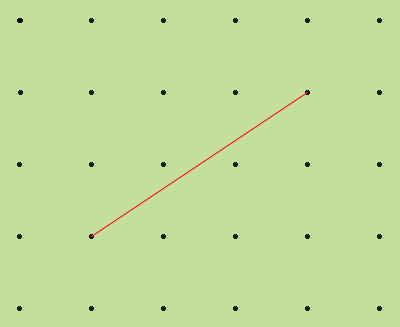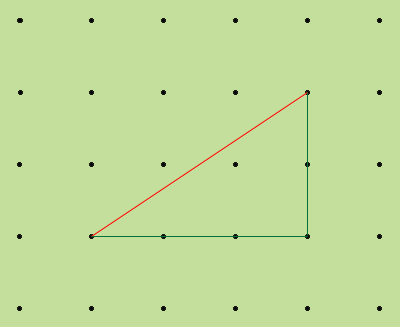SEARCH HOMEMath Central Quandaries & QueriesSubject: Pythagoras Name: Khaori Who are you: Student Hello, My name is Khaori and i am in 8th grade. I have this problem. I have to find the length of these lines that i have to find but i couldn't because i didn't know how. the problem reads: The three line segments below are drawn on centimeter dot paper. (the dot paper is a 5x6, the first line is a straight line and the length is two, there are two other lines but they are slanted and i don't know how to use Pythagoras theorem to find the length because i don't know were to get the numbers from) a. Find the length of each segment to the nearest ten-thousandth of a centimeter. b. Could these line segments be arranged to form a triangle? If no, explain why or why not. If yes, answer this question: could they form a right triangle? Explain why or why not.Khaori,

If the line segments join dots on the dot paper like in the diagram below then you can use Pythagoras' theorem to find their lengths.Draw two more line segments to form a right triangle as in the diagram below.In my diagram the legs of the right triangle have lengths 2 cm and 3 cm so the length of the hypotenuse, the original line segment, is

sqrt(22 + 32) = √13 cm

PennyMath Central is supported by the University of Regina and The Pacific Institute for the Mathematical Sciences.